Impact Factor 1.895 | CiteScore 2.24
More on impact ›

# Frontiers in Physics

## Original Research ARTICLE

Front. Phys., 24 November 2014 | https://doi.org/10.3389/fphy.2014.00070

# Nominally brittle cracks in inhomogeneous solids: from microstructural disorder to continuum-level scale

• Laboratoire SPHYNX, Service de Physique de l'État Condensé, DSM, Institut Rayonnement Matière de Saclay, CEA Saclay, CNRS URA 2464, Gif-sur-Yvette, France

We analyze the intermittent dynamics of cracks in heterogeneous brittle materials and the roughness of the resulting fracture surfaces by investigating theoretically and numerically crack propagation in an elastic solid of spatially-distributed toughness. The crack motion splits up into discrete jumps, avalanches, displaying scale-free statistical features characterized by universal exponents. Conversely, the ranges of scales are non-universal and the mean avalanche size and duration depend on the loading microstructure and specimen parameters according to scaling laws which are uncovered. The crack surfaces are found to be logarithmically rough. Their selection by the fracture parameters is formulated in term of scaling laws on the structure functions measured on one-dimensional roughness profiles taken parallel and perpendicular to the direction of crack growth.

## 1. Introduction

Understanding how solids break continues to pose significant fundamental challenges. For brittle solids broken under tension, Linear Elastic Fracture Mechanics (LEFM) tackles the difficulty by reducing the problem to the destabilization and subsequent growth of a dominant pre-existing crack (see e.g.,  for an introduction to LEFM). The theory is based on the idea that, in an elastic material, all dissipative and damaging processes are localized in a small zone around the crack tip, so-called fracture process zone, FPZ. Crack destabilization and further motion are then governed by the balance between the flux of mechanical energy released in the FPZ from the surrounding material and the dissipation rate into this zone. The former is computable within linear elasticity theory and connects to the stress intensity factor, which characterizes the near-tip stress field and depends on the external loading and specimen geometry only. The dissipation rate is quantified by the fracture energy required to expose a new unit area of cracked surfaces. Equivalently, it can be quantified by the fracture toughness, i.e., the onset value for the stress intensity factor above which crack starts to grow. Within LEFM theory, both the fracture energy and the fracture toughness are assumed to be material constants, to be measured experimentally.

LEFM framework provides a coherent framework to describe fracture in homogeneous solids. In contrast, heterogeneous solids remain unclear. Stress concentration at the crack tip makes the behavior observed at the continuum-level scale extremely sensitive to material's small scale inhomogeneities. Consequences include crackling dynamics for fracture with discrete pulses (avalanches) of a variety of sizes and erratic crack paths (see  for review). A generic observation in this field is the existence of scale free statistics: (i) Direct imaging of the crack motion in paper sheets [3, 4] or along heterogeneous interfaces [5, 6] has revealed power-law distribution for the size of the crack jumps; (ii) the acoustic  or seismic [10, 11] events going along with fracture are characterized by power-law distribution for the energy; and iii) fracture surfaces are found to exhibit self-affine morphological features  or logarithmic  roughness.

These observations, by essence, cannot be addressed by conventional LEFM continuum approaches. In this context, over the past 25 years, promising alternative approaches have emerged from statistical and non-linear physics: Statistical lattice models like fiber bundle models (see  for review) or random fuse models (see  for review), for example, were found to reproduce, with a minimal set of ingredients, crackling dynamics in failure  and self-affine fracture roughness , in qualitative agreement with some of the experimental observations. However, these approaches remain too minimal to provide quantitative predictions for situations of engineering interests.

A different approach consists in considering the crack propagation in a solid with spatially-distributed toughness. The availability of asymptotic formulas  for the variations of stress intensity factors along a slightly distorted crack can explicitly take into account the microstructural disorder in a continuum-level scale LEFM-like description . Within this framework, referred thereafter to as the Random-Toughness Continuum-Mechanics (RT-CM) approach, the fracture onset can be mapped to a critical depinning transition [2, 3335]. Depending on the situation, this approach yields logarithmically rough  or self-affine  fracture surfaces. It can also explain the crackling dynamics sometimes observed as a consequence of a self-adjustment of the driving force around the depinning value . The main advantage here is that the external parameters involved in the depinning model can be mapped to conventional LEFM parameters. This has been used to relate effective toughness and microstructural disorder [38, 39], or to unravel the specific conditions required to observed crackling in brittle fracture .

In the present work, depinning models unravel how the loading rate, specimen geometry, and microstructural disorder quantitatively select the statistics of continuum-level scale avalanches (as measured in conventional experimental fracture mechanics) and of the crack roughness (as recorded in conventional fractography). Section 2 presents the derivation of the depinning model from LEFM. This section details the successive assumptions and their implications. Section 3 assesses the statistics of the continuum-level scale avalanches, i.e., bursts evidenced in the time evolution of the spatially-averaged crack velocity. Statistics on the avalanche's size and duration demonstrate a power-law characterized by universal exponents. Conversely, the associated cutoffs do depend on the loading, microstructure, and specimen parameters according to scaling laws which are uncovered. Two regimes can be distinguished: A regime of pseudo-isolated avalanches not so different from what is predicted in the quasi-static limit (vanishing loading rate); and a regime where avalanches coalesce with each other. Section 4 analyzes the statistics of fracture roughness. The structure functions measured along profiles parallel and perpendicular to the direction of crack growth exhibit logarithmic scaling with prefactors and characteristic length-scales depending on the Poisson's ratio and microstructure parameters according to scaling which are uncovered.

## 2. Materials and Methods

In LEFM theory, the crack velocity v is governed by the balance between the mechanical energy G and the (material constant) fracture energy Γ (Griffith criterion). For slow cracks, v/μ = G − Γ where μ = cR/Γ relates the mobility μ to the Rayleigh wave speed cR. The principle of local symmetry (PLS) governs the growth direction . This imposes a zero stress intensity factor condition for mode II (KII = 0) all along the fracture. As consequences, an initially straight crack in an ideal homogeneous material gently loaded in mode I would continuously grow within its plane, without any jerky motion or roughness. But inhomogeneities at the microstructure scale yield distortions of the crack front, which, in turn, induce perturbations δKI, δKII, and δKIII in the local loading of the front.

In the RT-CM approach, the effect of material inhomogeneities is taken into account by introducing a random spatially-distributed component for the fracture energy and for the shear part of the loading. Then, Griffith criterion and PLS combined with an asymptotic estimation of the perturbations in the loading induced by the front distortion describes the crack growth. This approach was pioneered by Gao and Rice  and subsequently developed [3032, 37, 40, 4244]. Here, the model is reviewed with an emphasis on the relation between the model parameters and experimentally measurable quantities. We also pay special attention to list the various assumptions and discuss the implied limits.

### 2.1. From Front Distortions to the Perturbations in the Crack-Tip Loading

Let one consider a crack embedded in an isotropic elastic solid of size L × H × W under tension. In the following, we adopt the usual convention of fracture mechanics and the axes $\stackrel{{\to }}{{e}}$x, $\stackrel{{\to }}{{e}}$y and $\stackrel{{\to }}{{e}}$z align with the direction of crack propagation (L direction), tensile loading (H direction), and mean crack front (W direction), respectively. For a straight crack, the mode I stress intensity factor K0 fully characterizes the near-tip stress field loading. Let one now consider the situation depicted in Figure 1: left where the presence of inhomogeneities yields small in-plane and out-of-plane crack distortions, f(z, t) and h(x = f(z, t), z) respectively. The first step in the RT-CM approach is to estimate how these distortions perturb locally the loading. To make the problem tractable, simplifying hypothesis are needed:

Hyp. 1 The Young modulus E and Poisson ratio ν of the solid are considered as homogeneous.

Hyp. 2 The spatial distribution of Γ(x, y, z) and the PLS noise are narrow enough such that a first-order perturbation analysis can characterize the coupling of the local perturbations δKI(z), δKII(z) and δKIII(z) to the front distortions {f, h}.

FIGURE 1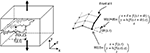Figure 1. Left: Sketch and notation of a crack front propagating in a heterogeneous solid. Right: Decomposition of the crack path into infinitesimal straight kinks.

Then, Rice  and Movchan et al's  formula relate δKi(z) to the front distortions:

where ∫ denotes a principal value integral. Note that δKI depends only on the in-plane distortions {f}, and δKII, δKIII depend on the out-of-plane distortions {h}. Here, several additional assumptions were required:

Hyp. 3 In the expressions of δKII and δKIII, we have omitted the terms brought by the non-singular stresses near the reference straight crack front (the so-called T-stress and the third term A of the asymptotic expansion of near-tip stress field). This assumption remains valid for stable crack paths (T ≤ 0 ) when the roughness wavelength is small with respect to the system size and the characteristic distances defined by the external loading [31, 36]. A complete form of the omitted terms can be found in Movchan et al. ;

Hyp. 4 Rice's formula for δKI assumes a sample with infinite width W and thickness H. It remains valid as long as one considers front corrugations the wavelength of which are small with respect to H and W. A more accurate formula proposed in Legrand et al.  addresses the case of finite H. To the very best of our knowledge, there does not exist any formula taking into account the effect of finite width, W.

### 2.2. Griffith Criterion and Equation of Motion

The above expression for the local perturbations δKi leads to a first order expression of the energy release rate G(z) as a function of the front distortions:

where G0(t, f) = K0(t, f)2/E is the reference energy release rate which, as K0(t, f), would result from the same loading with a straight crack front within the mean plane y = 0 at the mean position x = f(t). Henceforth, the operator a indicates averaging of the variable a(z) over the spatial coordinate z. The two quantities G0 and K0 coincide with the ones that would have been defined in the conventional LEFM approach when ignoring front distortions. They only depend on the specimen geometry and imposed load (of which both evolve with t) and can be determined using continuum mechanics (e.g., finite element analysis).

Slow cracking (as considered herein) implies that the solid is loaded by imposing external displacements rather than external stresses (the opposite would yield dynamic fracture with crack at speed on the order of the sound speed). This induces additional constraints: G0 should decrease with f(t) (specimen compliance always increases with crack length) and increase with t (to drive a crack, external displacement can only increase with time). Without loss of generality, we set t = 0 at a time when the crack has just stopped and x = 0 is the crack tip's position at this time. G0(t = 0, f = 0) = Γ where Γ = 〈Γ(x, y, z)〉 is the mean value of fracture energy. Considering the subsequent variation f(z, t) to be small with respect to the crack length at t = 0, we can write:

where Ġ = ∂G0/∂t (driving rate) and G′ = − ∂G0/∂f (unloading factor) are positive constants characterizing the loading rate and the specimen geometry, respectively. Inserting Equations 1 and 3 in Equation 2, and the resulting expression for G(z) into Griffith criterion, the equation of motion for the in-plane front displacement is:

where γ(x, y, z) = Γ(x, y, z) − Γ is the fluctuating part of the fracture energy. The solution of this equation provides the space-time dynamics of the in-plane projection of the crack front. Subsequently, it gives the time evolution of the fracture velocity at the continuum-level scale, v(t) = df/dt. This is the relevant observable characteristics of the crack dynamics in standard experiments of fracture mechanics.

### 2.3. Principle of Local Symmetry and Equation of Path

We now derive an equation of path by making use of the principle of local symmetry . To do so, we take inspiration from the work of Katzav et al. [44, 47] to model crack path in a model 2D situation and extend it for three-dimensional solids. The idea is to decompose the front propagation along x-axis into infinitesimal straight kinks of length ℓ, identified with the microstructural length-scale characterizing the spatial distribution of Γ(x, y, z) (see Figure 1:right). Consider now the kink occurring at the front location z between time t and t+d t. Leblond et al's formulas  relate the stress intensity factors after kinking Ki(z) (i = I, II, III) to the stress intensity factors before kinking Ki(z) and to the kink angle δθ = θ(z, t + dt) − θ(z, t) = ℓ ∂2h/∂x2(x = f(z, t), z) via:

where Fi,j(δθ) are universal functions that were computed in Leblond  for three-dimensional solids. The principle of local symmetry implies that KII must be uniformly zero, which leads to:

Now, to first order in δθ, FII, I(δθ) and FII, II(δθ) are given by Leblond : FII, I(δθ) = δθ + O(δθ2) and FII, II(δθ) = 1 + O(δθ2). The kink angle is then related to K0, δKI(z) and δKII(z) via: δθ ≈ −δKII(z)/(K0 + δKI(z)). Finally, by keeping only the first order loading perturbations, it writes:

To introduce the effect of microstructural disorder, a spatially distributed noise term K0ϑ (x, y, z) is added to the right-handed side of the equation. In the situation of inter-granular crack growth in a material made of sintered grains (e.g. sandstone), for example, this additional term would translate the difference between the kink angle predicted by the principle of local symmetry and that truly selected so that the crack propagates between the cemented grains. Finally, combining the resulting equation with the asymptotic formula for stress intensity factors (Equation 1), one gets:

The solution of this equation provides the topography of the post-mortem fracture surfaces, h(x, z). This is the quantity of interest in fractography science. Note that this equation differs from that given in Larralde  and Bonamy et al.  as it includes an additional curvature term ∂2h/∂x2.

### 2.4. Relevant Parameters and Numerical Aspects

Equations 4 and 8 predict deterministically the fracture dynamics and the morphology of fracture surfaces using the following inputs:

Input 1 The loading rate Ġ;

Input 2 The specimen geometry (unloading factor G′ and specimen width W)

Input 3 The material constants (fracture energy Γ, Poisson ratio ν, and mobility μ),

Input 4 The microstructure disorder (microstructure length-scale ℓ and the spatial distribution of the two noise terms γ(x, y, z) and ϑ(x, y, z)).

Statistically, the two noise terms are characterized by the probability density functions p(γ) and p(ϑ) and the spatial correlation functions Cγ($\stackrel{{\to }}{{r}}$) = 〈γ($\stackrel{{\to }}{{r}}$0 + r)γ($\stackrel{{\to }}{{r}}$0)〉$\stackrel{{\to }}{{r}}$0 and Cϑ($\stackrel{{\to }}{{r}}$) = 〈γ($\stackrel{{\to }}{{r}}$0 + r)γ($\stackrel{{\to }}{{r}}$0)〉$\stackrel{{\to }}{{r}}$0. Additional assumptions simplify the problem:

Hyp. 5 The two noise terms are not correlated; At first glance, such an assumption may appear odd since both terms originate from the material heterogeneities. But due to the tensorial nature of elasticity, these heterogeneities will affect the equation of motion and the equation of path independently. As an illustrative example, let us consider again the situation of intergranular crack growth in a solid made of sintered grains. Two distinct space-dependent noise terms are required to describe the microstructural texture: The first one quantifies the local variations of adhesion between grains and mainly affects the equation of motion; the second one describes the local variations of joint orientation and affects the equation of path.

Hyp. 6 Both probability density functions are Gaussian of zero mean and standard deviation $\stackrel{{˜}}{{\gamma }}$ and $\stackrel{{˜}}{{\theta }}$, respectively;

Hyp. 7 In both cases, the correlation function C decreases linearly with |r| over the microstructure distance ℓ and is zero above.

Note that, in elastic interfaces equations as that proposed in Equations 4 and 8, the scaling properties remain unaffected upon changes in the probability functions (p(γ) and p(ϑ)) and in the correlation functions (Cγ(|r|) and Cϑ(|r|)) . Thanks to this series of assumptions, the two spatially distributed noise terms are fully characterized with 3 parameters: $\stackrel{{˜}}{{\gamma }}$, $\stackrel{{˜}}{{\theta }}$ and ℓ.

At this point, the Equations 4 and 8 are coupled via the two 3D noise terms γ(x, y, z) and ϑ(x, y, z), since they both depend on the in-plane and out-of-plane positions of the crack front. A last assumption permits the decoupling of the two equations:

Hyp. 8 The 3D spatially distributed terms γ(x, y, z) and ϑ(x, y, z) reduce to their in-plane projection γ(x, z) and ϑ(x, z).

As shown in Ramanathan et al. , Equation 8 with a 2D projection for the noise term yields logarithmically smooth fracture surfaces. Hence, zooming out on the fracture surfaces makes them appear flatter and flatter. When the zooming out is sufficient, fluctuations in h become negligible when compared to in-plane length scales. The two noise terms reduce to γ(x, y = h(x, z), z) ≈ γ(x, y = 0, z) and ϑ(x, y = h(x, z), z) ≈ ϑ(x, y = 0, z). This validates Hyp. 8.

At this point, the selection of the fracture behavior brings into play 8 parameters: μ, Γ, Ġ, G′, $\stackrel{{˜}}{{\gamma }}$, $\stackrel{{˜}}{{\theta }}$, ℓ and W. The introduction of dimensionless time tt/ℓ/(μΓ) and length {x, y, z, f, h} → {x/ℓ, y/ℓ, z/ℓ, f/ℓ, h/ℓ}) reduces this number. Equations 4 and 8 become:

where the dimensionless noise term η is characterized by a standard deviation $\stackrel{{˜}}{{\eta }}$ = $\stackrel{{˜}}{{\gamma }}$/Γ and a spatial correlation length equal to unity.

The final two decoupled dimensionless equations require only 6 parameters:

• The dimensionless driving rate c = Ġℓ/μΓ2,

• the dimensionless unloading factor k = G′ℓ/Γ,

• the Poisson-ratio dependent parameter A = (2 − 3ν)/(2 − ν),

• the parameters $\stackrel{{˜}}{{\eta }}$ and $\stackrel{{˜}}{{\theta }}$ characterizing the disorder strength,

• the continuum-level vs. microstructure scale ratio N = W/ℓ.

The following sections subsequently address the continuum-level scale statistics of crack dynamics v(t) and the morphology of the post-mortem fracture surfaces h(x, z). They also reveal their dependencies on the external parameters {c, k, A, $\stackrel{{˜}}{{\eta }}$, $\stackrel{{˜}}{{\theta }}$, N}. The numerical scheme is the following. For each set of parameters, we built two uncorrelated random N × pN maps η(x, z) and ϑ(x, z) with Gaussian distribution of variance $\stackrel{{˜}}{{\eta }}$ and $\stackrel{{˜}}{{\theta }}$, respectively. At time t = 0, the crack front is a straight line at x = 0: f(z, t = 0) = 0 and h(x = 0, z) = 0 ∀ z ∈ [0, N − 1]. Solving Equation 9a gives the space-time evolution of f(z, t). Solving Equation 9b provides h(x, z). Both cases invoke a fourth order Runge-Kutta scheme and periodic boundary conditions along z: f(z = 0, t) = f(z = N, t) and h(x, z = 0) = h(x, z = N) for all t and x. This speeds up the computation time as the two integral terms in the equations can be solved in the Fourier domain.

## 3. Results

### 3.1. Crackling Dynamics and Avalanche Statistics

We first look at the crack dynamics. Equation 9a describes the motion of a long-range elastic chain driven in a frozen random pinning potential with a driving force F = ctkf(t) self-adjusting around the depinning value . For {c, k} → 0, the chain propagates while remaining at the critical depinning point and the crack moves through irregular jumps, or avalanches. The size S and duration D of the avalanche1 follow a universal power-law distribution [37, 49]: P(S) ∝ S−τ and P(D) ∝ D−α where the exponents τ and α can be estimated using renormalization  or numerical [52, 53] methods. The most precise estimations yields τ = 1.280 ± 0.01 and α = 1.500 ± 0.010 . These scale free statistics extend to upper cutoffs S0 and D0 set by the system size N: S0Nζ and D0Nκ where ζ and κ are the roughness exponent and dynamic exponent, respectively. The most precise estimations of their value yield [52, 53]: ζ = 0.385 ± 005 and κ = 0.770 ± 0.005.

Here, both c and k are finite. The effects of a finite unloading factor k keeping c → 0 is now fairly well documented [2, 54]: It modifies the upper cutoffs S0 and D0 to the preceding scale-free distributions:

Here, the form of the functions f(u) and g(u) is expected to be universal. The exponents 1/σ and 1/Δ are also predicted to be universal: 1/σ = 0.69 ± 0.010 and 1/Δ = 0.385 ± 0.010 [2, 54]. Conversely, the effect of a finite c is not uncovered yet. By yielding some overlap between the avalanches , it can significantly alter the dynamics. In particular, a recent work  has evidenced a transition line between a crackling-like dynamics made of irregular power-law distributed jumps and a continuum-like dynamics ruled by the conventional LEFM theory.

Figure 2 presents typical times profiles of the continuum-level scale velocity v(t) for different values of c and k in the crackling regime. Here and thereafter, the system size N and the disorder strength $\stackrel{{˜}}{{\eta }}$ are set constant, N = 1024 and $\stackrel{{˜}}{{\eta }}$ = 1, returning at the end of this section to a brief discussion on their effect. Note the irregular jumps characteristics of the underlying avalanching dynamics. Note also the qualitative changes in the signal appearance as k and c are modulated: Pulses become shorter as k increases, and the pulse density increases as c increases. Note finally that, due to the finite value of c, v(t) does not vanish between the pulse, but becomes equal to a small value proportional to c (prefactor dependent of the Runge Kunta scheme). The avalanches are then identified with the bursts where v(t) is above a prescribed reference level vc = 10−3. Their duration D is defined as the interval between the two successive intersections of v(t) with vc, and their size S is defined as the integral of v(t) − vc between the same points.

FIGURE 2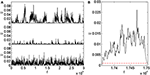Figure 2. (A) Evolutions of the continuum-level scale velocity v(t) for three typical parameter sets within the crackling phase: {c = 10−5, k = 10−3, $\stackrel{{˜}}{{\eta }}$ = 1, N = 1024} (top), {c = 10−5, k = 10−2, $\stackrel{{˜}}{{\eta }}$ = 1, N = 1024} (middle), {c = 10−4, k = 10−2, $\stackrel{{˜}}{{\eta }}$ = 1, N = 1024} (bottom). (B) Zoom in on an avalanche, identified as a pulse where v(t) is above a prescribed value vc = 10−3 (horizontal red dash line). The avalanche duration D is the interval between the two successive intersections of v(t) with vc. The avalanche size S is the integral of v(t) − vc between the same points.

Figure 3 reports the probability density function of avalanche size P(S|c, k) and duration P(D|c, k) for different values of c and k. Power-laws are observed. The exponents τ and α associated with the power-law decrease are independent of c and k. Moreover, they compare well with the values τ = 1.280 ± 0.01 and α = 1.500 ± 0.010 expected for {c, k} → 0. On the other hand, the valid region of the power-law is observed depends on c and k. Both the lower cutoffs Smin and Dmin are roughly independent of c and k. However, the precise values Smin and Dmin were observed to depend on the reference level vc and, thus, are to be associated with the procedure to extract the avalanches. We will consider in the following only the part of the distributions above these lower cutoffs.

FIGURE 3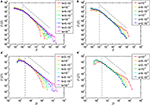Figure 3. Top: Distribution of the avalanche size measured (A) for various values of k at constant c = 10−4 and (B) for various values of c at constant k = 10−2. In both cases, N = 1024 and $\stackrel{{˜}}{{\eta }}$ = 1 and the axes are logarithmic. The power-law exponent τ is found to be independent of the parameters and compatible to the universal value τ = 1.28 (black dashed line) predicted for c → 0 and k → 0. The lower cutoff is found to be independent of the parameters: Smin ≈ 10−3 (vertical dash line). The upper-cutoff is found to decrease with k and to increase with c (resp. to be independent of c) when c is large enough (resp. when c is small enough). Bottom: Distribution of the avalanche duration measured (A′) for various values of k at constant c = 10−4 and (B′) for various values of c at constant k = 10−2. In both cases, N = 1024 and $\stackrel{{˜}}{{\eta }}$ = 1 and the axes are logarithmic. The power-law exponent α is found to be independent of the parameters and compatible to the universal value α = 1.50 (black dashed line) predicted for c → 0 and k → 0. The lower cutoff is found to be independent of the parameters: Dmin ≈ 3 (vertical dash line). The upper-cutoff is found to decrease with k and to increase with c (resp. to be independent of c) when c is large enough (resp. when c is small enough).

The power-law distributions observed in Figure 3 also exhibit upper cutoffs S0 and D0. These cutoffs decrease with k in all cases. The effects of c is of two types:

• For large k/small c the cutoff does not depend on c (only on k).

• At small k/large c, the cutoff increases with c and the distribution also displays a bump at large sizes and durations.

Direct computation of the cutoff is quite imprecise. Hence, the selection of the typical length and time scales is studied via variations of the mean values 〈S〉(c, k) = ∫Smin S × P(S|c, k)dS (Figure 4A) and 〈D〉 = ∫Dmin D × P(D|c, k)dD (Figure 4B). At large enough k, both 〈S〉 and 〈D〉 are independent of c. This large k regime is attributed to a regime of pseudo-isolated (pi) avalanches. The distributions are then expected to take forms similar to that of Equation 10. As a result, 〈Spi is expected  to take the form 〈SpiSτ − 1minS2 − τ0k−(2 − τ)/σ, 〈DpiDα − 1minD2 − α0k−(2 − α)/Δ. These two scaling are compatible with the observations at large k (dash line in Figures 4A,B). As a synthesis, the mean avalanche size and duration are found to take the following form at large k:

FIGURE 4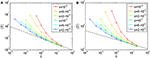Figure 4. (A) Evolution of the mean avalanche size 〈S〉 as a function of the unloading factor k for different loading rates c. The curves collapse for large k onto a c-independent power-law curve. Black thick dash line depicts a fit over the collapsed region using Equation 11a: 〈Spi ≈ 10−2 k−(2 − τ)/σ with τ = 1.28 and 1/σ = 0.69. (B) Evolution of the mean avalanche duration 〈D〉 as a function of the unloading factor k for different value of the loading rate c. At large k, the curves collapse onto a c-independent power-law curve. Black thick dash line is a fit over the collapsed region using Equation 11b : 〈Dpi ≈ 11k−(2 − α)/Δ with α = 1.50 and 1/Δ = 0.385. In both (A,B) N = 1024 and $\stackrel{{˜}}{{\eta }}$ = 1 and the axes are logarithmic. The different symbols correspond to different values of c, given in the legends.

Let us try now to characterize the effects of the avalanche overlap when k becomes small or c becomes large. Previous work  evidenced a transition between the crackling dynamics studied here and a continuum-like dynamics when c becomes large enough or k small enough. This transition is believed  to coincide with the point where the avalanche overlap percolates throughout the entire system. At constant $\stackrel{{˜}}{{\eta }}$ and N, this transition was shown  to be fully driven by the ratio c/k. We hence plotted, in Figure 5, 〈S〉/〈Spi and 〈D〉/〈Dpi (first order estimation of the number of individual avalanches having merged together to form the bursts detected from the signal v(t)), as a function of the control parameter c/k. A coarse collapse is observed. As expected, the master curves diverge at the transition value between crackling and continuum-like dynamics (materialized by the vertical dash lines in the main panels of Figures 5A,B).

FIGURE 5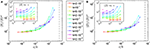Figure 5. (A) Main panel: Variation of 〈S〉/〈Spi as a function of c/k for different k. (A) Inset: 〈S〉 as a function of c for different k. (B) Main panel: Variation of 〈D〉/〈Dpi as a function of c/k for different k. (B) Inset: 〈D〉 as a function of c for different k. In both (A,B) N = 1024 and $\stackrel{{˜}}{{\eta }}$ = 1 and the axes are logarithmic. The different symbols correspond to different values of k, given in the legend between graphs (A,B). In the main panels of both graphs, the vertical dash lines indicates the transition value c/k between crackling and continuum-like dynamics as determined in Nukala et al.  for N = 1024 and $\stackrel{{˜}}{{\eta }}$ = 1.

Note that significant deviations to the collapse are observed. They are believed to stem from a qualitative change in the distribution shape as k decreases/c increases and the avalanche overlap increases. Actually, for small k/large c a bump develops at large scales, for both the distribution in size and in duration. Due to this change in shape, several length-scales (resp. time-scales) intervenes in the distributions of S (resp. D). Thus, the recording of the mean values alone is not sufficient to capture their evolutions with c and k. Ongoing work aims at characterizing these effects more accurately.

To make the analysis complete, we looked at the effects of the system size N and disorder strength $\stackrel{{˜}}{{\eta }}$. Figure 6 plots the probability density function of avalanche size P(S|N, $\stackrel{{˜}}{{\eta }}$) and avalanche duration P(D|N, $\stackrel{{˜}}{{\eta }}$) as measured for different values of N and $\stackrel{{˜}}{{\eta }}$ at fixed values of c and k. For both size and duration, the decrease of N yields an increase of the lower-cutoffs Smin and Dmin (main panel of Figures 6A,A′). The two dependencies are well fitted by power-laws: SminNaSN and DminNaDN with aSN ≈ 1.7 and aDN ≈ 0.6 (inset of Figures 6A,A′). $\stackrel{{˜}}{{\eta }}$ does not seems to affect Smin. Conversely, Dmin decreases with $\stackrel{{˜}}{{\eta }}$ as Dmin$\stackrel{{˜}}{{\eta }}$aD$\stackrel{{˜}}{{\eta }}$ with aD$\stackrel{{˜}}{{\eta }}$ ≈ 1.2 (inset of Figure 6B′). The effects of N and $\stackrel{{˜}}{{\eta }}$ of 〈S〉 and 〈D〉 are analyzed in Figure 7, by plotting the curves 〈S〉 vs c and 〈D〉 vs c for different N at constant k and $\stackrel{{˜}}{{\eta }}$ (insets in Figures 7A,A′), and for different $\stackrel{{˜}}{{\eta }}$ at constant k and N (insets in Figures 7B,B′). Increasing N yields a decrease of the low-c plateau and a leftward shift of the divergence location for both 〈S〉 and 〈D〉. Increasing $\stackrel{{˜}}{{\eta }}$ yields an increase of the low-c plateau for 〈S〉, a decrease of the low-c plateau for 〈D〉, and a rightward shift of the divergence location for both 〈S〉 and 〈D〉. All curves can then be superimposed by making {〈S〉 → 〈S〉/NbSN, cc/NcN} in the main panel of Figure 7A, {〈S〉 → 〈S〉/$\stackrel{{˜}}{{\eta }}$bS$\stackrel{{˜}}{{\eta }}$, cc/$\stackrel{{˜}}{{\eta }}$c$\stackrel{{˜}}{{\eta }}$} in the main panel of Figure 7B, {〈D〉 → 〈D〉/NbDN, cc/NcN} in the main panel of Figure 7A′, {〈D〉 → 〈D〉/$\stackrel{{˜}}{{\eta }}$bD$\stackrel{{˜}}{{\eta }}$, cc/$\stackrel{{˜}}{{\eta }}$c$\stackrel{{˜}}{{\eta }}$} in the main panel of Figure 7B′. By combining this with Equation 11 and the collapse obtained in Figure 5, we anticipate the following form for mean size and duration: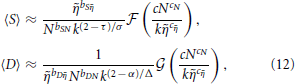where the two functions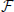(u) and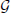(u) exhibit a plateau at small u, and both diverge at the same value uc. The value of the exponents τ, α, 1/σ and 1/Δ are well known : They can e.g., be related to the so-called roughness exponent ζ and dynamic exponent κ classically defined in the realm of critical depinning transition: τ = 2 − 1/(1 + ζ), α = 1 + ζ/κ, 1/σ = (1 + ζ)/2 and 1/Δ = κ/(1 + ζ). Conversely, the precise origin of the exponents bSN, bS$\stackrel{{˜}}{{\eta }}$, bDN, bD$\stackrel{{˜}}{{\eta }}$, cN, and c$\stackrel{{˜}}{{\eta }}$ and their link with ζ and κ remain to be uncovered.

FIGURE 6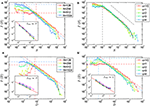Figure 6. Effect of the system size N and disorder strength $\stackrel{{˜}}{{\eta }}$ on the avalanche statistics. Main panel, top: Distribution of the avalanche size measured (A) for different N at constant c = 2 × 10−5, k = 10−2 and $\stackrel{{˜}}{{\eta }}$ = 1, and (B) for different $\stackrel{{˜}}{{\eta }}$ at constant c = 2 × 10−5, k = 10−2 and N = 1024. The axes are logarithmic. Main panel, bottom: Distribution of the avalanche duration measured (A′) for different N at constant c = 2 × 10−5, k = 10−2 and $\stackrel{{˜}}{{\eta }}$ = 1, and (B′) for different $\stackrel{{˜}}{{\eta }}$ at constant c = 2 × 10−5, k = 10−2 and N = 1024. All axes are logarithmic. In all panels, the power-law exponents τ and α are compatible with the universal values τ = 1.28 and α = 1.50 (inclined dashed line) predicted for c → 0 and k → 0. Regarding the size, the lower cutoff Smin is observed to be independent of $\stackrel{{˜}}{{\eta }}$ and to decrease with N. Regarding the duration, the lower cutoff Dmin decreases with both N and $\stackrel{{˜}}{{\eta }}$. Smin (resp. Dmin) is quantitatively defined as the intersection of the power-law regime with exponent τ (resp. α) and the saturation value for P observed at small S (resp. small D). The red and blue dash lines in (A,A′,B′) present illustrations for N = 128 and N = 1024, respectively. The variation of Smin with N is shown in the inset of a. The variations of Dmin with N and $\stackrel{{˜}}{{\eta }}$ are shown in the insets of (A′,B′). The different symbols correspond to different values for c (from 2 × 10−6 to 10−4). The red line are fits: SminNaSN with aSN = 1.7 ± 0.1, DminNaDN with aDN = 0.6 ± 0.1, and Dmin$\stackrel{{˜}}{{\eta }}$aD$\stackrel{{˜}}{{\eta }}$ with aD$\stackrel{{˜}}{{\eta }}$ = 1.2 ± 0.1.

FIGURE 7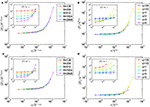Figure 7. Effect of the system size N and disorder strength $\stackrel{{˜}}{{\eta }}$ on the mean size and duration of avalanches. Top, inset: Mean avalanche size 〈S〉 as a function of c for constant k = 10−2 and (A) different N at constant $\stackrel{{˜}}{{\eta }}$ = 1 and (B) different $\stackrel{{˜}}{{\eta }}$ at constant N = 1024. Bottom, inset: Mean avalanche duration 〈D〉 as a function of c and constant k = 10−2 and (A′) different N and constant $\stackrel{{˜}}{{\eta }}$ = 1 and (B′) different $\stackrel{{˜}}{{\eta }}$ and constant N = 1024. In all cases, the axes are logarithmic. Main panels: Curve collapse obtained by plotting 〈S〉/NbSN vs c/N−cN (A), 〈S〉/$\stackrel{{˜}}{{\eta }}$bS$\stackrel{{˜}}{{\eta }}$ vs c/Nc$\stackrel{{˜}}{{\eta }}$ (B), 〈D〉/N−bDN vs c/N−cN (A′), and 〈D〉/$\stackrel{{˜}}{{\eta }}$bD$\stackrel{{˜}}{{\eta }}$ vs c/N−c$\stackrel{{˜}}{{\eta }}$. The fitted exponents are found to be bSN = 1.3 ± 0.1, bS$\stackrel{{˜}}{{\eta }}$ = 0.7 ± 0.1, bDN = 0.45 ± 0.1, bD$\stackrel{{˜}}{{\eta }}$ = 0.65 ± 0.1, cN = 0.65 ± 0.1, c$\stackrel{{˜}}{{\eta }}$ = 1.05 ± 0.1

Equation 12 quantitatively relate the material parameters to quantities that are accessible in conventional experimental mechanics, namely the mean size and duration of the avalanches. In this context, it is of interest to rewrites the equation with the original variables, before the non-dimensionalization procedure: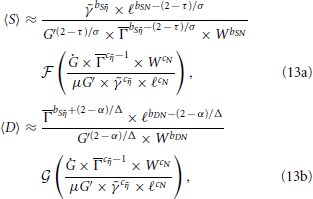where the mean avalanche size 〈S〉 and duration 〈D〉 are expressed with real length and time units, and Ġ, G′, W, Γ, μ, ℓ and $\stackrel{{˜}}{{\gamma }}$ are recalled to be the loading rate, the unloading factor, the specimen width, the fracture energy, the mobility, the microstructure length-scale, and the contrast in local fracture energy. The value of the exponents are recalled to be τ = 1.280 ± 0.01 (predicted), α = 1.500 ± 0.01 (predicted), 1/σ = 0.69 ± 0.01 (predicted), 1/Δ = 0.385 ± 0.01 (predicted), bSN = 1.3 ± 0.1 (fitted), bS$\stackrel{{˜}}{{\eta }}$ = 0.7 ± 0.1 (fitted), bDN = 0.45 ± 0.1 (fitted), bD$\stackrel{{˜}}{{\eta }}$ = 0.65 (fitted), cN = 0.65 ± 0.1 (fitted), and c$\stackrel{{˜}}{{\eta }}$ = 1.05 ± 0.1 (fitted).

### 3.2. Roughness of Fracture Surfaces

We turn now to the topography h(x, z) of the post-mortem fracture surfaces as predicted by equation 9b. Figure 8 reports typical topographies for different values of the two external parameters A and $\stackrel{{˜}}{{\theta }}$. When A is close to 1, the surface seems to be statistically isotropic while as A gets smaller, the surface appears more elongated in the direction of z. Conversely, the parameter $\stackrel{{˜}}{{\theta }}$ only affects the range swept by the roughness. Note that in almost all elastic solids, Poisson ratio ν lies between 0 and 0.5, which impose a finite interval for A = (2 − 3ν)/(2 − ν): 1/3 ≤ A ≤ 1. Herein, only A within this interval are considered.

FIGURE 8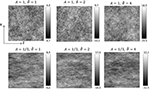Figure 8. Typical topographies h(x, z) obtained by solving Equation 9b for different values of A and ϑ. For each case, the image size is 1024 × 1024 and the height ranges over the colorbar indicated on the right. Here, the x and z axes coincide with the vertical and the horizontal, respectively.

To characterize quantitatively the spatial distribution of fracture roughness, we adopted the classical procedure  and computed the structure function S$\stackrel{{\to }}{{r}}$) = 〈(h($\stackrel{{\to }}{{r}}$ + Δ$\stackrel{{\to }}{{r}}$) − h($\stackrel{{\to }}{{r}}$))2〉. Here, the operator 〈〉 denotes averaging over all positions $\stackrel{{\to }}{{r}}$ = (x, z). First, we computed the structure function Szz) along 1D profiles taken parallel to z (mean direction of the crack front). The procedure is the following: (i) an initially straight front was first propagated over a distance equal to 10N to obtain a statistically stationary regime; (ii) 10000 subsequent profiles h(xi, z) separated by a distance xi+1xi = 1 were recorded; (iii) the structure function Sizz) was computed for each of these profiles; and (iv) finally, these 10000 individual structure functions were averaged to get Szz).

Figure 9 depicts typical examples of Szz) curves for different values of N, θ and A. Sz goes as Sz = pzlog(Δzz) up to an upper cutoff set by the system size N. This logarithmic scaling is anticipated to extend over the whole range of length-scales as N → ∞. Note that logarithmically rough crack surfaces were also predicted in earlier theoretical works [31, 32] analyzing crack propagation through a three-dimensional heterogeneous solid. As a plus, the present model allows relating the prefactor pz and characteristic length-scale λz with the fracture parameters: pz is found to scale as $\stackrel{{˜}}{{\theta }}$2/A (Figure 10A), while λz is independent of both A and $\stackrel{{˜}}{{\theta }}$ (Figure 10B). Finally, the structure function along z is:

FIGURE 9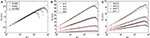Figure 9. Structure function Szz) measured along z: (A) for various system sizes N at A = 1 and $\stackrel{{˜}}{{\theta }}$ = 1, (B) for various $\stackrel{{˜}}{{\theta }}$ at A = 1 and N = 1024, and (C) for various A at $\stackrel{{˜}}{{\theta }}$ = 1 and N = 1024. The ordinate axis is logarithmic. For all figures, the straight lines correspond to the solutions of Equation 14.

FIGURE 10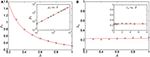Figure 10. (A) Slope pz associated with the curve Sz vs. Δz as a function of A at $\stackrel{{˜}}{{\theta }}$ = 1 (main), and as a function of $\stackrel{{˜}}{{\theta }}$ at A = 1 (inset). In the inset, the axes are logarithmic. In both graphs, the red lines correspond to fits pz = C/A (main) and pz = C$\stackrel{{˜}}{{\theta }}$2 where C = 0.32 ± 0.02. (B) Characteristic length-scale λz associated with the curve Sz vs. Δz as a function of A at constant $\stackrel{{˜}}{{\theta }}$ = 1 (main) and as a function of $\stackrel{{˜}}{{\theta }}$ at constant A = 1 (inset). In both graphs, the red lines correspond to fits λz = 0.24 ± 0.03. Here, ± indicates a 95% confident interval.

We now look at the structure function Sxx) along x. A direct computation of Sx following the standard procedure proposed for Sz was found to give a large scattering even for a constant set of parameters {N, A, $\stackrel{{˜}}{{\theta }}$}. Hence, the computation procedure was modified as follows: (i) an initially straight front was first propagated over a distance equal to 10N to obtain a statistically stationary regime; (ii) the x evolution of $\stackrel{{˜}}{{h}}$(x, zi) = h(x, zi) − h(x) was subsequently recorded at 100 locations zi uniformly distributed along the specimen width N (h(x) denotes averaging over the specimen width N); (iii) the structure function Sizx) = 〈($\stackrel{{˜}}{{h}}$(x + Δx, zi) − $\stackrel{{˜}}{{h}}$(x, zi))2〉 was computed for each of these profiles; (iv) these 100 individual structure functions were averaged to get the Sxx) for a single specimen; and (v) the so-obtained structure functions were further averaged over 100 specimens. This procedure produces accurate and reproducible curves Sx vs. x.

Figure 11 depicts typical examples of Sxx) curves for different values of N, θ and A. The behavior resembles that observed for Sz, with a logarithmic scaling Sx = pxlog(Δxx) up to an upper cutoff set by the system size N. Note that Sx saturates above the cutoff, and does not decrease down to zero as was observed for Sz due to periodic boundary conditions. As for Sz, the prefactor (now referred to as px) goes as $\stackrel{{˜}}{{\theta }}$2/A (Figure 12A). The characteristic length-scale (λx) is independent of $\stackrel{{˜}}{{\theta }}$ (Figure 12B:inset). But contrary to what is observed for Sz, this characteristic length λx depends on A: λx ∝ 1/A. This dependency is responsible for the apparent stretching along s of the images in Figure 10 observed as A decreases. Finally, the structure function along x is:

FIGURE 11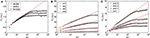Figure 11. Structure function Sxx) measured along x (A) for various system sizes N at A = 1 and $\stackrel{{˜}}{{\theta }}$ = 1, (B) for various $\stackrel{{˜}}{{\theta }}$ at A = 1 and N = 1024, and (C) for various A at constant $\stackrel{{˜}}{{\theta }}$ = 1 and N = 1024. The ordinate axis is logarithmic. For all figures, the straight lines correspond to the solutions of Equation 15.

FIGURE 12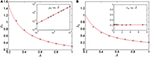Figure 12. (A) Slope px associated with the curve Sx vs. Δx as a function of A at constant $\stackrel{{˜}}{{\theta }}$ = 1 (main) and $\stackrel{{˜}}{{\theta }}$ at constant A = 1 (inset). In the inset, the axes are logarithmic. In both graphs, the red lines are fits p1 = Cx/A (main) and p1 = C$\stackrel{{˜}}{{\theta }}$2where the fitted parameter is found to be C = 0.31 ± 0.02 (95% confident interval). (B) Characteristic length-scale λx associated with the curve Sx vs. Δx as a function of A at constant $\stackrel{{˜}}{{\theta }}$ = 1 (main) and $\stackrel{{˜}}{{\theta }}$ at constant A = 1 (inset). In both graphs, the red lines are fits λx = 0.21 ± 0.02/A (main) and λx = 0.21 ± 0.02 (inset). Here, ± indicates a 95% confident interval.

Equations 14 and 15 quantitatively relate the material parameters (microstructure and Poisson ratio) to quantities accessible in conventional fractography analysis. In this context, it is of interest to rewrites them with the original variables, before the non-dimensionalization procedure:

where Sx, Sz, Δx and Δz are expressed with real length units, and ν, ℓ and $\stackrel{{˜}}{{\theta }}$ are recalled to be the Poisson ratio, the microstructure scale, and disorder contrast.

## 4. Concluding Discussion

Stress enhancement at crack tips makes the macroscale failure behavior observed extremely sensitive to the presence of disorder at the microstructure scale. This translates into crackling dynamics and rough fracture surfaces, which, by essence, cannot be addressed within the conventional LEFM framework. In this paper, we have used the RT-CM approach to obtain quantitative relations between some statistical observables characteristic of these two aspects and the fracture parameters: Loading rate (time derivative of the energy release rate), specimen geometry (specimen thickness and unloading factor), conventional mechanical constants (fracture energy, Poisson ratio), and microstructural disorder (microstructure scale and disorder strength).

Over a certain range of the fracture parameters, this RT-CM approach predicts crackling dynamics : The crack growth splits up into discrete jumps, which are power-law distributed in size and duration. The characteristic exponents associated to these power-laws are universal. Conversely, the scales covered by these scale-free features are non-universal and, in particular, the mean size and duration of the crack jumps are found to depend on the fracture parameters according to scaling laws that are uncovered. These scaling laws can be understood over a certain range of the fracture parameters, in the regime of pseudo-isolated avalanches addressable via standard functional renormalization theory [49, 5153]. Conversely, the effect of the avalanche overlapping is not understood. On-going work aims at analyzing the distribution of the local avalanches as detected in the space-time diagrams of the front dynamics, in order to understand the coalescence process.

Also, this RT-CM approach predicts rough fracture surfaces. The fracture roughness can be characterized by computing the structure function, which exhibits logarithmic scaling. The associated prefactor and characteristic length-scale are found to depend on the Poisson ratio, microstructure length-scale, and disorder strength according to laws that were uncovered. This may have interesting applications: It allows one to infer the microstructure parameters (the access of which could be made difficult otherwise, due to the smallness of the length-scales involved) from the analysis of post-mortem fracture surfaces at larger scale. Work in progress aims at testing the scaling predicted here for the structure functions against fractography experiments achieved in oxide glasses.

Note finally that the RT-CM model studied here call upon a variety of assumptions (see Section 2). An interesting perspective would be to measure to which extend these assumptions can be released. Work in this direction is currently under progress. The model is also limited to nominally brittle fracture, with a single macroscopic crack propagating in an otherwise intact material. Promising alternative approaches have emerged from statistical and non-linear physics [18, 57] and may permit to address quasi-brittle fracture, with many microcracking events interacting with each-others. A central challenge in the field would be to bridge the gap between these approaches and engineering damage mechanics.

## Conflict of Interest Statement

The authors declare that the research was conducted in the absence of any commercial or financial relationships that could be construed as a potential conflict of interest.

## Acknowledgments

The authors would like to thank Alberto Rosso for enlightening discussions and the “Agence Nationale de la Recherche” (ANR) for financial support of the project MEPHYSTAR (ANR-09-SYSC-006-01).

## Footnotes

1. ^In problems of depinning of interfaces, the definition of the avalanche size differ depending on the publication. Sometimes, the size is defined as the area A swept by the front between two successive depinning configurations. Here, the size S is defined as the integral of the continuum-level scale velocity v(t) between the two successive depinning configurations. The two definitions are related by S = A/N when N is the system size.

## References

1. Lawn B. Fracture of brittle solids (Cambridge Solide State Science). Cambridge, UK: Cambridge University Press. (1993). doi: 10.1017/CBO9780511623127

2. Bonamy D. Intermittency and roughening in the failure of brittle heterogeneous materials. J Phys D Appl Phys. (2009) 42:214014. doi: 10.1088/0022-3727/42/21/214014

3. Santucci S, Vanel L, Ciliberto S. Subcritical statistics in rupture of fibrous materials: experiments and model. Phys Rev Lett. (2004) 93:095505. doi: 10.1103/PhysRevLett.93.095505

4. Stojanova M, Santucci S, Vanel L, Ramos O. High frequency monitoring reveals aftershocks in subcritical crack growth. Phys Rev Lett. (2014) 112:115502. doi: 10.1103/PhysRevLett.112.115502

5. Måløy KJ, Santucci S, Schmittbuhl J, Toussaint R. Local waiting time fluctuations along a randomly pinned crack front. Phys Rev Lett. (2006) 96:045501. doi: 10.1103/PhysRevLett.96.045501

6. Tallakstad KT, Toussaint R, Santucci S, Schmittbuhl J, Måloy KJ. Local dynamics of a randomly pinned crack front during creep and forced propagation: an experimental study. Phys Rev E (2011) 83:046108. doi: 10.1103/PhysRevE.83.046108

7. Petri A, Paparo G, Vespignani A, Alippi A, Costantini M. Experimental evidence for critical dynamics in microfracturing processes. Phys Rev Lett. (1994) 73:3423. doi: 10.1103/PhysRevLett.73.3423

8. Garcimartin A, Guarino A, Bellon L, Ciliberto S. Statistical properties of fracture precursors. Phys Rev Lett. (1997) 79:3202–5. doi: 10.1103/PhysRevLett.79.3202

9. Baro J, Corral A, Illa X, Planes A, Salje EKH, Schranz W, et al. Statistical similarity between the compression of a porous material and earthquakes. Phys Rev Lett. (2013) 110:088702. doi: 10.1103/PhysRevLett.110.088702

10. Bak P, Christensen K, Danon L, Scanlon T. Unified scaling law for earthquakes. Phys Rev Lett. (2002) 88:178501. doi: 10.1103/PhysRevLett.88.178501

11. Corral A. Long-term clustering, scaling, and universality in the temporal occurrence of earthquakes. Phys Rev Lett. (2004) 92:108501. doi: 10.1103/PhysRevLett.92.108501

12. Mandelbrot BB, Passoja DE, Paullay AJ. Fractal character of fracture surfaces of metals. Nature (1984) 308:721–2. doi: 10.1038/308721a0

13. Bouchaud E, Lapasset G, Planès J. Fractal dimension of fractured surfaces: a universal value? Europhys Lett. (1990) 13:73–9.

Google Scholar

14. Måløy KJ, Hansen A, Hinrichsen EL, Roux S. Experimental measurements of the roughness of brittle cracks. Phys Rev Lett. (1992) 68:213–5. doi: 10.1103/PhysRevLett.68.213

15. Ponson L, Bonamy D, Bouchaud E. 2d scaling properties of experimental fracture surfaces. Phys Rev Lett. (2006) 96:035506. doi: 10.1103/PhysRevLett.96.035506

16. Ponson L, Auradou H, Pessel M, Lazarus V, Hulin JP. Failure mechanisms and surface roughness statistics of fractured fontainebleau sandstone. Phys Rev E (2007) 76:036108. doi: 10.1103/PhysRevE.76.036108

17. Dalmas D, Lafarge A, Vandembroucq D. Crack propagation through phase separated glasses: effect of the characteristic size of disorder. Phys Rev Lett. (2008) 101:255501. doi: 10.1103/PhysRevLett.101.255501

18. Alava MJ, Nukala PKVV, Zapperi S. Statistical models of fracture. Adv Phys. (2006) 55:349–476. doi: 10.1080/00018730300741518

19. Hansen A, Hemmer PC. Burst avalanches in bundles of fibers - local versus global load-sharing. Phys Lett A (1994) 184:394–6. doi: 10.1016/0375-9601(94)90511-8

20. Zapperi S, Nukala PKVV, Simunovic S. Crack roughness and avalanche precursors in the random fuse model. Phys Rev E (2005) 71(2 Pt 2):026106. doi: 10.1103/PhysRevE.71.026106

21. Danku Z, Kun F. Temporal and spacial evolution of bursts in creep rupture. Phys Rev Lett. (2013) 111:084302. doi: 10.1103/PhysRevLett.111.084302

22. Hansen A, Hinrichsen H, Roux S. Roughness of crack interfaces. Phys Rev Lett. (1991) 66:2476–9. doi: 10.1103/PhysRevLett.66.2476

23. Nukala PKVV, Zapperi S, Simunovic S. Crack surface roughness in three-dimensional random fuse networks. Phys Rev E (2006) 74(2 Pt 2):026105. doi: 10.1103/PhysRevE.74.026105

24. Nukala PKVV, Barai P, Zapperi S, Alava MJ, Simunovic S. Fracture roughness in three-dimensional beam lattice systems. Phys Rev E (2010) 82:026103. doi: 10.1103/PhysRevE.82.026103

25. Rice JR. 1st-order variation in elastic fields due to variation in location of a planar crack front. J Appl Mech. (1985) 52:571–9. doi: 10.1115/1.3169103

26. Movchan A, Gao H, Willis J. On perturbations of plane cracks. Int J Solids Struct. (1998) 35:3419–53. doi: 10.1016/S0020-7683(97)00231-X

27. Leblond JB. Crack paths in three-dimensional elastic solids. i: two-term expansion of the stress intensity factors – application to crack path stability in hydraulic fracturing. Int J Solids Struct. (1999) 36:79–103. doi: 10.1016/S0020-7683(97)00276-X

28. Lazarus V. Perturbation approaches of a planar crack in linear elastic fracture mechanics: a review. JMPS (2011) 59:121. doi: 10.1016/j.jmps.2010.12.006

29. Gao H, Rice JR. A first order perturbation analysis on crack trapping by arrays of obstacles. J Appl Mech. (1989) 56:828. doi: 10.1115/1.3176178

30. Schmittbuhl J, Roux S, Vilotte JP, Måløy KJ. Interfacial crack pinning: effect of nonlocal interactions. Phys Rev Lett. (1995) 74:1787–90. doi: 10.1103/PhysRevLett.74.1787

31. Larralde H, Ball RC. The shape of slowly growing cracks. Europhys Lett. (1995) 30:87–92. doi: 10.1209/0295-5075/30/2/005

32. Ramanathan S, Ertas D, Fisher DS. Quasistatic crack propagation in heterogeneous media. Phys Rev Lett. (1997) 79:873. doi: 10.1103/PhysRevLett.79.873

33. Roux S, Vandembroucq D, Hild F. Effective toughness of heterogeneous brittle materials. Eur J Mech A (2003) 22:743–9. doi: 10.1016/S0997-7538(03)00078-0

34. Ponson L. Depinning transition in the failure of inhomogeneous brittle materials. Phys Rev Lett. (2009) 103:055501. doi: 10.1103/PhysRevLett.103.055501

35. Bonamy D, Bouchaud E. Failure of heterogeneous materials: a dynamic phase transition? Phys Rep. (2011) 498:1–44. doi: 10.1016/j.physrep.2010.07.006

36. Bonamy D, Ponson L, Prades S, Bouchaud E, Guillot C. Scaling exponents for fracture surfaces in homogeneous glass and glassy ceramics. Phys Rev Lett. (2006) 97:135504. doi: 10.1103/PhysRevLett.97.135504

37. Bonamy D, Santucci S, Ponson L. Crackling dynamics in material failure as the signature of a self-organized dynamic phase transition. Phys Rev Lett. (2008) 101:045501. doi: 10.1103/PhysRevLett.101.045501

38. Patinet S, Vandembroucq D, Roux S. Quantitative prediction of effective toughness at random heterogeneous interfaces. Phys Rev Lett. (2013) 110:165507. doi: 10.1103/PhysRevLett.110.165507

39. Démery V, Rosso A, Ponson L. From microstructural features to effective toughness in disordered brittle solids. EPL (2014) 105:34003. doi: 10.1209/0295-5075/105/34003

40. Barés J, Barbier L, Bonamy D. Crackling versus continuumlike dynamics in brittle failure. Phys Rev Lett. (2013) 111:054301. doi: 10.1103/PhysRevLett.111.054301

41. Gol'dstein RV, Salganik R. Brittle fracture of solids with arbitrary cracks. Int J Fract. (1974) 10:507–23. doi: 10.1007/BF00155254

42. Charles Y, Vandembroucq D, Hild F, Roux S. Material-independent crack arrest statistics. J Mech Phys Solids (2004) 52:1651–69. doi: 10.1016/j.jmps.2003.12.004

43. Vandembroucq D, Skoe R, Roux S. Universal depinning force fluctuations of an elastic line: application to finite temperature behavior. Phys Rev E (2004) 70:051101. doi: 10.1103/PhysRevE.70.051101

44. Katzav E, Adda-Bedia M. Stability and roughness of tensile cracks in disordered materials. Phys Rev E (2013) 88:052402. doi: 10.1103/PhysRevE.88.052402

45. Cotterell B, Rice JR. Slighly curved or kinked cracks. Int J Fract. (1980) 16:155–69. doi: 10.1007/BF00012619

46. Legrand L, Patinet S, Leblond JB, Frelat J, Lazarus V, Vandembroucq D. Coplanar perturbation of a crack lying on the mid-plane of a plate. Int J Fract. (2011) 170:67–82. doi: 10.1007/s10704-011-9603-0

47. Katzav E, Adda-Bedia M, Derrida B. Fracture surfaces of heterogeneous materials: a 2d solvable model. Europhys Lett. (2007) 78:46006. doi: 10.1209/0295-5075/78/46006

48. Leblond JB, Lazarus V, Mouchrif S. Crack paths in three-dimensional elastic solids. ii: three-term expansion of the stress intensity factors – applications and perspectives. Int J Solids Struct. (1999) 36:105–42. doi: 10.1016/S0020-7683(97)00271-0

49. Ertas D, Kardar M. Critical dynamics of contact line depinning. Phys Rev E (1994) 49:R2532. doi: 10.1103/PhysRevE.49.R2532

50. Chauve P, Doussal PL, Wiese KJ. Renormalization of pinned elastic systems: how does it work beyond one loop? Phys Rev Lett. (2001) 86:1785. doi: 10.1103/PhysRevLett.86.1785

51. Dobrinevski A, Doussal PL, Wiese KJ. Statistics of avalanches with relaxation, and barkhausen noise. Phys Rev E (2013) 88:032106. doi: 10.1103/PhysRevE.88.032106

52. Rosso A, Krauth W. Roughness at the depinning threshold for a long-range elastic string. Phys Rev E (2002) 65:025101. doi: 10.1103/PhysRevE.65.025101

53. Duemmer O, Krauth W. Depinning exponents of the driven long-range elastic string. J Stat Mech. (2007) 1:01019. doi: 10.1088/1742-5468/2007/01/P01019

54. Laurson L, Santucci S, Zapperi S. Avalanches and clusters in planar crack front propagation. Phys Rev E (2010) 81:046116. doi: 10.1103/PhysRevE.81.046116

55. White RA, Dahmen KA. Driving rate effects on crackling noise. Phys Rev Lett. 91:085702. doi: 10.1103/PhysRevLett.91.085702

56. LeDoussal P, Wiese KJ. Size distributions of shocks and static avalanches from the functional renormalization group. Phys Rev E (2009) 79:051106. doi: 10.1103/PhysRevE.79.051106

57. Pradhan S, Hansen A, Chakrabarti BK. Failure processes in elastic fiber bundles. Rev Mod Phys. (2010) 82:499. doi: 10.1103/RevModPhys.82.499

Keywords: fracture, solid mechanics, crackling, fractals, scaling laws

Citation: Barés J, Barlet M, Rountree CL, Barbier L and Bonamy D (2014) Nominally brittle cracks in inhomogeneous solids: from microstructural disorder to continuum-level scale. Front. Phys. 2:70. doi: 10.3389/fphy.2014.00070

Received: 04 August 2014; Accepted: 05 November 2014;
Published online: 24 November 2014.

Edited by:

Ferenc Kun, University of Debrecen, Hungary

Reviewed by:

Mikko Alava, Aalto University, Finland
Osvanny Ramos, University Claude Bernard Lyon 1, France
Eytan Katzav, Hebrew University in Jerusalem, Israel

Copyright © 2014 Barés, Barlet, Rountree, Barbier and Bonamy. This is an open-access article distributed under the terms of the Creative Commons Attribution License (CC BY). The use, distribution or reproduction in other forums is permitted, provided the original author(s) or licensor are credited and that the original publication in this journal is cited, in accordance with accepted academic practice. No use, distribution or reproduction is permitted which does not comply with these terms.

*Correspondence: Daniel Bonamy, Laboratoire SPHYNX, Service de Physique de l'État Condensé, DSM, Institut Rayonnement Matière de Saclay, CEA Saclay, CNRS URA 2464, Batiment 772, Orme des Merisiers, F-91191 Gif sur Yvette Cedex, France e-mail: daniel.bonamy@cea.fr